Dot Situation Quiz I, Non Verbal Reasoning

# Dot Situation Quiz I, Non Verbal Reasoning - Police Constable Exams

Test Description

## 15 Questions MCQ Test General Intelligence & Reasoning for Police Exams - Dot Situation Quiz I, Non Verbal Reasoning

Dot Situation Quiz I, Non Verbal Reasoning for Police Constable Exams 2023 is part of General Intelligence & Reasoning for Police Exams preparation. The Dot Situation Quiz I, Non Verbal Reasoning questions and answers have been prepared according to the Police Constable Exams exam syllabus.The Dot Situation Quiz I, Non Verbal Reasoning MCQs are made for Police Constable Exams 2023 Exam. Find important definitions, questions, notes, meanings, examples, exercises, MCQs and online tests for Dot Situation Quiz I, Non Verbal Reasoning below.
Solutions of Dot Situation Quiz I, Non Verbal Reasoning questions in English are available as part of our General Intelligence & Reasoning for Police Exams for Police Constable Exams & Dot Situation Quiz I, Non Verbal Reasoning solutions in Hindi for General Intelligence & Reasoning for Police Exams course. Download more important topics, notes, lectures and mock test series for Police Constable Exams Exam by signing up for free. Attempt Dot Situation Quiz I, Non Verbal Reasoning | 15 questions in 30 minutes | Mock test for Police Constable Exams preparation | Free important questions MCQ to study General Intelligence & Reasoning for Police Exams for Police Constable Exams Exam | Download free PDF with solutions
 1 Crore+ students have signed up on EduRev. Have you?
Dot Situation Quiz I, Non Verbal Reasoning - Question 1

### Directions to Solve From amongst the figures marked (1), (2), (3) and (4), select the figure which satisfies the same conditions of placement of the dots as in figure (X). Question - Select the figure which satisfies the same conditions of placement of the dots as in Figure-X.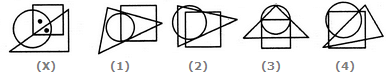Detailed Solution for Dot Situation Quiz I, Non Verbal Reasoning - Question 1
In fig. (X), one of the dots lies in the region common to the circle and the square only and the other dot lies in the region common to all the three figures -the circle, the square and the triangle. In each of the alternatives (1), (2) and (3), there is no region common to the square and the circle only. Only fig. (4) consists of both the types of regions.
Dot Situation Quiz I, Non Verbal Reasoning - Question 2

### Directions to Solve From amongst the figures marked (1), (2), (3) and (4), select the figure which satisfies the same conditions of placement of the dots as in figure (X). Question - Select the figure which satisfies the same conditions of placement of the dots as in Figure-X.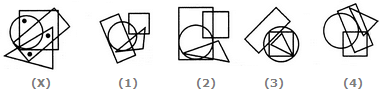Detailed Solution for Dot Situation Quiz I, Non Verbal Reasoning - Question 2
In fig. (X), one of the dots lies in the region common to the circle and the square only, another dot lies in the region common to the square, the triangle and the rectangle only and the third dot lies in the region common to the triangle and the rectangle only. In each of the figures (1), (2) and (3) there is no region common to the square, the triangle and the rectangle only. Only fig. (4) consists of all the three types of regions.
Dot Situation Quiz I, Non Verbal Reasoning - Question 3

### Directions to Solve From amongst the figures marked (1), (2), (3) and (4), select the figure which satisfies the same conditions of placement of the dots as in figure (X). Question - Select the figure which satisfies the same conditions of placement of the dots as in Figure-X.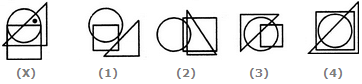Detailed Solution for Dot Situation Quiz I, Non Verbal Reasoning - Question 3
In fig. (X), the dot is contained in the region common to the triangle and the circle only. Out of the four alternatives, only fig. (3) contains a region common to the triangle and the circle only.
Dot Situation Quiz I, Non Verbal Reasoning - Question 4

From amongst the figures marked (1), (2), (3) and (4), select the figure which satisfies the same conditions of placement of the dots as in figure (X).

Question -

Select the figure which satisfies the same conditions of placement of the dots as in Figure-X.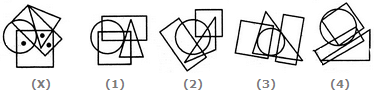Detailed Solution for Dot Situation Quiz I, Non Verbal Reasoning - Question 4
In fig. (X), one of the dots lies in the region common to the circle and the square only, another dot lies in the region common to the square, the triangle and the rectangle only and the third dot lies in the region common to the square and the rectangle only. In fig. (2) there is no region common to the square and the rectangle only, in fig. (3) there is no region common to the circle and the square only and in fig. (4) there is no region common to the square, the triangle and the rectangle only. Only fig. (1) consists of all the three types of regions.
Dot Situation Quiz I, Non Verbal Reasoning - Question 5

From amongst the figures marked (1), (2), (3) and (4), select the figure which satisfies the same conditions of placement of the dots as in figure (X).

Question -

Select the figure which satisfies the same conditions of placement of the dots as in Figure-X.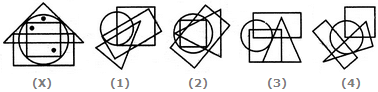Detailed Solution for Dot Situation Quiz I, Non Verbal Reasoning - Question 5
In fig. (X), one of the dots lies in the region common to the circle and the triangle only, another dot lies in the region common to the circle, the square and the triangle only and the third dot lies in the region common to the circle, the square and the rectangle only. In each of the figures (1) and (3) there is no region common to the circle and the triangle only. In fig. (4) there is no region common to the circle, the square and the rectangle only. Only fig. (2) consists of all the three types of regions.
Dot Situation Quiz I, Non Verbal Reasoning - Question 6

From amongst the figures marked (1), (2), (3) and (4), select the figure which satisfies the same conditions of placement of the dots as in figure (X).

Question -

Select the figure which satisfies the same conditions of placement of the dots as in Figure-X.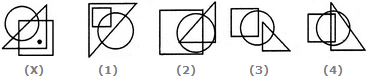Detailed Solution for Dot Situation Quiz I, Non Verbal Reasoning - Question 6
In fig. (X), the dot is contained in the region common to the triangle and the square only. Out of the four alternatives, only fig. (1) contains a region common to the triangle and the square only.
Dot Situation Quiz I, Non Verbal Reasoning - Question 7

From amongst the figures marked (1), (2), (3) and (4), select the figure which satisfies the same conditions of placement of the dots as in figure (X).

Question -

Select the figure which satisfies the same conditions of placement of the dots as in Figure-X.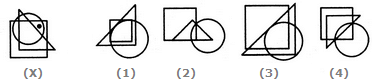Detailed Solution for Dot Situation Quiz I, Non Verbal Reasoning - Question 7
In fig. (X), the dot is contained in the region common to the square and the circle only. Out of the four alternatives, only fig. (4) contains a region common to the square and the circle only.
Dot Situation Quiz I, Non Verbal Reasoning - Question 8

From amongst the figures marked (1), (2), (3) and (4), select the figure which satisfies the same conditions of placement of the dots as in figure (X).

Question -

Select the figure which satisfies the same conditions of placement of the dots as in Figure-X.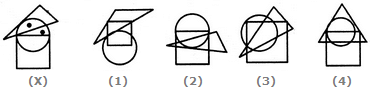Detailed Solution for Dot Situation Quiz I, Non Verbal Reasoning - Question 8
In fig. (X), one of the dots lies in the region common to the circle and the triangle only and the other dot lies in the circle alone. In each of the two figures (1) and (2), there is no region common to the circle and the triangle only. In fig. (4) there is no region which lies in the circle alone. Only fig. (3) consists of both the types of regions.
Dot Situation Quiz I, Non Verbal Reasoning - Question 9

From amongst the figures marked (1), (2), (3) and (4), select the figure which satisfies the same conditions of placement of the dots as in figure (X).

Question -

Select the figure which satisfies the same conditions of placement of the dots as in Figure-X.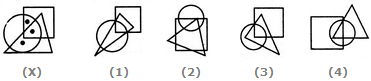Detailed Solution for Dot Situation Quiz I, Non Verbal Reasoning - Question 9
In fig. (X), one of the dots lies in the region common to the circle and the triangle only, another dot lies in the region common to all the three figures - the circle, the square and the triangle and the third dot lies in the region common to the circle and the square only. In each of the figures (1) and (3), there is no region common to the circle and the square only and in fig. (2), there is no region common to the circle and the triangle only. Only fig. (4) consists of all the three types of regions.
Dot Situation Quiz I, Non Verbal Reasoning - Question 10

From amongst the figures marked (1), (2), (3) and (4), select the figure which satisfies the same conditions of placement of the dots as in figure (X).

Question -

Select the figure which satisfies the same conditions of placement of the dots as in Figure-X.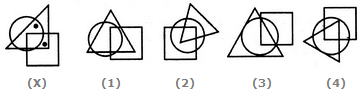Detailed Solution for Dot Situation Quiz I, Non Verbal Reasoning - Question 10
In fig. (X), one of the dots is placed in the region common to the circle and the triangle only and another dot is placed in the region common to the square and the triangle only. In each of the three alternatives (2), (3) and (4), there is no region common to the square and the triangle only. Only fig. (1) consists of both the types of regions.
Dot Situation Quiz I, Non Verbal Reasoning - Question 11

From amongst the figures marked (1), (2), (3) and (4), select the figure which satisfies the same conditions of placement of the dots as in figure (X).

Question -

Select the figure which satisfies the same conditions of placement of the dots as in Figure-X.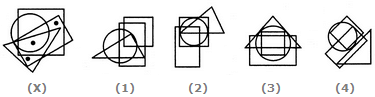Detailed Solution for Dot Situation Quiz I, Non Verbal Reasoning - Question 11
In fig. (X), one of the dots lies in the region common to the square and the rectangle only, another dot lies in the region common to all the four elements - the circle, the square, the triangle and the rectangle and the third dot lies in the region common to the triangle and the rectangle only. In fig. (2) there is no region common to the triangle and the rectangle only. In fig. (3) there is no region common to the square and the rectangle only. In fig. (4) there is no region common to all the four elements - the circle, the square, the triangle and the rectangle. Only fig. (1) consists of all the three types of regions.
Dot Situation Quiz I, Non Verbal Reasoning - Question 12

From amongst the figures marked (1), (2), (3) and (4), select the figure which satisfies the same conditions of placement of the dots as in figure (X).

Question -

Select the figure which satisfies the same conditions of placement of the dots as in Figure-X.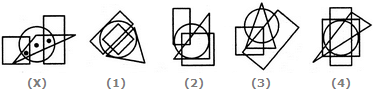Detailed Solution for Dot Situation Quiz I, Non Verbal Reasoning - Question 12
In fig. (X), one of the dots lies in the region common to the circle the triangle and the rectangle only, another dot lies in the region common to the circle and the triangle only and the third dot lies in the region common to the circle, the square and the triangle only. In each of the figures (1), (2) and (4) there is no region common to the circle, the triangle and the rectangle only. Only fig. (3) consists of all the three types of regions.
Dot Situation Quiz I, Non Verbal Reasoning - Question 13

From amongst the figures marked (1), (2), (3) and (4), select the figure which satisfies the same conditions of placement of the dots as in figure (X).

Question -

Select the figure which satisfies the same conditions of placement of the dots as in Figure-X.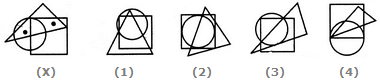Detailed Solution for Dot Situation Quiz I, Non Verbal Reasoning - Question 13
In fig. (X), one of the dots lies in the region common to the circle and the triangle only and the other dot lies in the region common to the square and the triangle only. In each of the figures (2), (3) and (4), there is no region common to the circle and the triangle only. Only fig. (1) consists of both the types of regions.
Dot Situation Quiz I, Non Verbal Reasoning - Question 14

From amongst the figures marked (1), (2), (3) and (4), select the figure which satisfies the same conditions of placement of the dots as in figure (X).

Question -

Select the figure which satisfies the same conditions of placement of the dots as in Figure-X.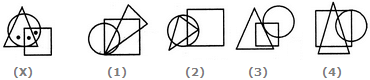Detailed Solution for Dot Situation Quiz I, Non Verbal Reasoning - Question 14
In fig. (X), one of the dots lies in the region common to the circle and the square only, another dot lies in the region common to all the three figures - the circle, the square and the triangle and the third dot lies in the region common to the circle and the triangle only. In each of the alternatives (1), (3) and (4), there is no region common to the circle and the triangle only. Only fig. (2) consists of all the three types of regions.
Dot Situation Quiz I, Non Verbal Reasoning - Question 15

From amongst the figures marked (1), (2), (3) and (4), select the figure which satisfies the same conditions of placement of the dots as in figure (X).

Question -

Select the figure which satisfies the same conditions of placement of the dots as in Figure-X.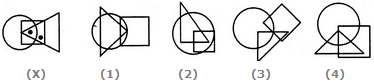Detailed Solution for Dot Situation Quiz I, Non Verbal Reasoning - Question 15
In fig. (X), one of the dots lies in the region common to the square and the triangle only and the other dot lies in the region common to all the three figures - the circle, the square and the triangle. In each of the alternatives (1) and (2), there is no region common to the square and the triangle only. In alternative (3), there is no region common to all the three figures. Only, alternative (4) consists of both the types of regions.

## General Intelligence & Reasoning for Police Exams

83 videos|44 docs|96 tests
Information about Dot Situation Quiz I, Non Verbal Reasoning Page
In this test you can find the Exam questions for Dot Situation Quiz I, Non Verbal Reasoning solved & explained in the simplest way possible. Besides giving Questions and answers for Dot Situation Quiz I, Non Verbal Reasoning, EduRev gives you an ample number of Online tests for practice

## General Intelligence & Reasoning for Police Exams

83 videos|44 docs|96 tests